Simplification And Approximation-MCQ Test

# Simplification And Approximation-MCQ Test

Test Description

## 10 Questions MCQ Test UPSC Prelims Paper 2 CSAT - Quant, Verbal & Decision Making | Simplification And Approximation-MCQ Test

Simplification And Approximation-MCQ Test for Banking Exams 2023 is part of UPSC Prelims Paper 2 CSAT - Quant, Verbal & Decision Making preparation. The Simplification And Approximation-MCQ Test questions and answers have been prepared according to the Banking Exams exam syllabus.The Simplification And Approximation-MCQ Test MCQs are made for Banking Exams 2023 Exam. Find important definitions, questions, notes, meanings, examples, exercises, MCQs and online tests for Simplification And Approximation-MCQ Test below.
Solutions of Simplification And Approximation-MCQ Test questions in English are available as part of our UPSC Prelims Paper 2 CSAT - Quant, Verbal & Decision Making for Banking Exams & Simplification And Approximation-MCQ Test solutions in Hindi for UPSC Prelims Paper 2 CSAT - Quant, Verbal & Decision Making course. Download more important topics, notes, lectures and mock test series for Banking Exams Exam by signing up for free. Attempt Simplification And Approximation-MCQ Test | 10 questions in 20 minutes | Mock test for Banking Exams preparation | Free important questions MCQ to study UPSC Prelims Paper 2 CSAT - Quant, Verbal & Decision Making for Banking Exams Exam | Download free PDF with solutions
 1 Crore+ students have signed up on EduRev. Have you?
Simplification And Approximation-MCQ Test - Question 1

### In a regular week, there are 5 working days and for each day, the working hours are 8. A man gets Rs. 2.40 per hour for regular work and Rs. 3.20 per hours for overtime. If he earns Rs. 432 in 4 weeks, then how many hours does he work for ?

Detailed Solution for Simplification And Approximation-MCQ Test - Question 1

Suppose the man works overtime for x hours.

Now, working hours in 4 weeks = (5 x 8 x 4) = 160.

∴ 160 x 2.40 + x x 3.20 = 432

⇒ 3.20x = 432 - 384 = 48

⇒ x = 15.

Hence, total hours of work = (160 + 15) = 175.

Simplification And Approximation-MCQ Test - Question 2

### Free notebooks were distributed equally among children of a class. The number of notebooks each child got was one-eighth of the number of children. Had the number of children been half, each child would have got 16 notebooks. Total how many notebooks were distributed ?

Detailed Solution for Simplification And Approximation-MCQ Test - Question 2

Let total number of children be x.

Then, x x(1/8)x = (x/2)x 16   ⇔  x = 64

Number of notebooks =(1/8)x2 =(1/8x 64 x 64)= 512

Simplification And Approximation-MCQ Test - Question 3

### A man has some hens and cows. If the number of heads be 48 and the number of feet equals 140, then the number of hens will be:

Detailed Solution for Simplification And Approximation-MCQ Test - Question 3

Let the number of hens be x and the number of cows be y.

Then, x + y = 48 .... (i)

and 2x + 4y = 140   ⇒   x + 2y = 70 .... (ii)

Solving (i) and (ii) we get: x = 26, y = 22.

∴ The required answer = 26.

Simplification And Approximation-MCQ Test - Question 4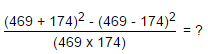Detailed Solution for Simplification And Approximation-MCQ Test - Question 4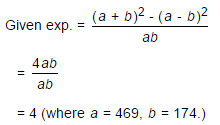Simplification And Approximation-MCQ Test - Question 5

David gets on the elevator at the 11th floor of a building and rides up at the rate of 57 floors per minute. At the same time, Albert gets on an elevator at the 51st floor of the same building and rides down at the rate of 63 floors per minute. If they continue travelling at these rates, then at which floor will their paths cross ?

Detailed Solution for Simplification And Approximation-MCQ Test - Question 5

Suppose their paths cross after x minutes.

Then, 11 + 57x = 51 - 63x    ⇔    120x = 40

x =1/3

Number of floors covered by David in (1/3) min. =((1/3)x 57)= 19

So, their paths cross at (11+19) i.e., 30th floor.

Simplification And Approximation-MCQ Test - Question 6

A sum of Rs. 1360 has been divided among A, B and C such that A gets (2/3) of what B gets and B gets (1/4) of what C gets. B's share is:

Detailed Solution for Simplification And Approximation-MCQ Test - Question 6

Let C's share = Rs. x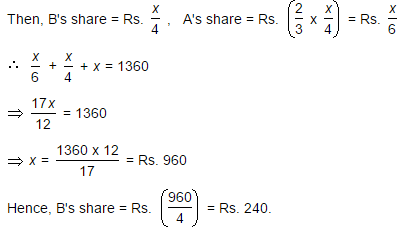Simplification And Approximation-MCQ Test - Question 7

One-third of Rahul's savings in National Savings Certificate is equal to one-half of his savings in Public Provident Fund. If he has Rs. 1,50,000 as total savings, how much has he saved in Public Provident Fund ?

Detailed Solution for Simplification And Approximation-MCQ Test - Question 7

Let savings in N.S.C and P.P.F. be Rs. x and Rs. (150000 - x) respectively. Then,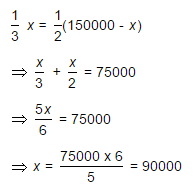Savings in Public Provident Fund = Rs. (150000 - 90000) = Rs. 60000

Simplification And Approximation-MCQ Test - Question 8

A fires 5 shots to B's 3 but A kills only once in 3 shots while B kills once in 2 shots. When B has missed 27 times, A has killed:

Detailed Solution for Simplification And Approximation-MCQ Test - Question 8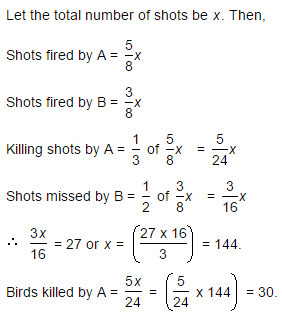Simplification And Approximation-MCQ Test - Question 9

Eight people are planning to share equally the cost of a rental car. If one person withdraws from the arrangement and the others share equally the entire cost of the car, then the share of each of the remaining persons increased by:

Detailed Solution for Simplification And Approximation-MCQ Test - Question 9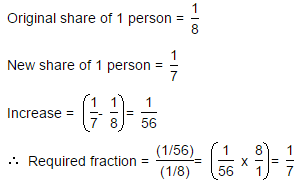Simplification And Approximation-MCQ Test - Question 10

To fill a tank, 25 buckets of water is required. How many buckets of water will be required to fill the same tank if the capacity of the bucket is reduced to two-fifth of its present ?

Detailed Solution for Simplification And Approximation-MCQ Test - Question 10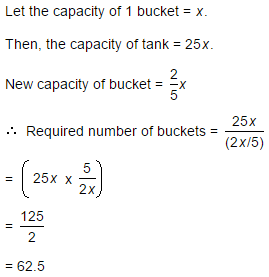## UPSC Prelims Paper 2 CSAT - Quant, Verbal & Decision Making

67 videos|50 docs|151 tests
 Use Code STAYHOME200 and get INR 200 additional OFF Use Coupon Code
Information about Simplification And Approximation-MCQ Test Page
In this test you can find the Exam questions for Simplification And Approximation-MCQ Test solved & explained in the simplest way possible. Besides giving Questions and answers for Simplification And Approximation-MCQ Test, EduRev gives you an ample number of Online tests for practice

## UPSC Prelims Paper 2 CSAT - Quant, Verbal & Decision Making

67 videos|50 docs|151 tests